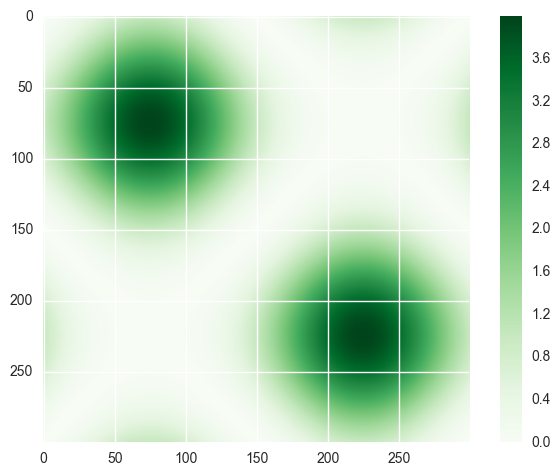# Basic example¶

This example notebook demonstrates as a basic example how sphinx-nbexamples works.

You can edit the metadata of the notebook as shown in the screenshot below and include supplementary files, select an image for displaying it in the gallery or provide a code example.Otherwise you can do whatever you want. You can also include ipython magic commands, e.g %matplotlib inline

In :
%matplotlib inline


which then will be (as well as all the warnings) removed in the rendered html file of your example.

By default, the sphinx-nbexamples looks for pictures you created with matplotlib and saved it in the notebook. If such a picture exists, it uses the last created picture as a thumbnail in the gallery. For this example, we modified the the thumbnail_figure key of the notebook metadata to use our own picture (the one you see above).

In :
import matplotlib.pyplot as plt
import numpy as np
import seaborn as sns

In :
x = np.linspace(-np.pi, np.pi, 300)

xx, yy = np.meshgrid(x, x)
z = (np.sin(xx) + np.sin(yy)) ** 2

plt.figure()
plt.imshow(z, cmap='Greens')
plt.colorbar()

Out:
<matplotlib.colorbar.Colorbar at 0x112349bd0>